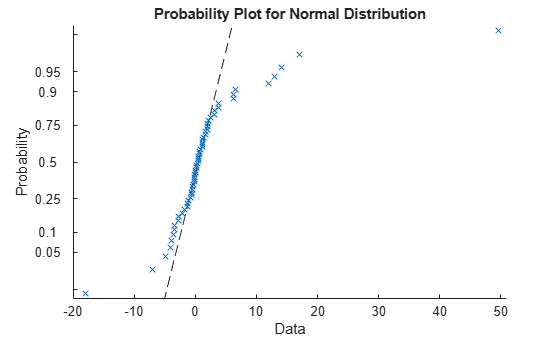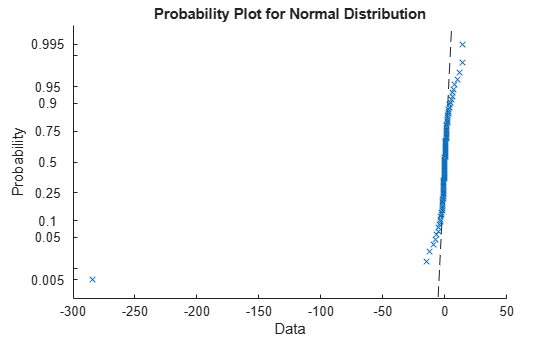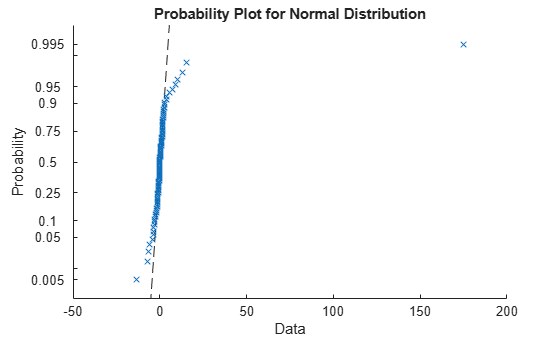# trimmean

Mean, excluding outliers

## Syntax

``m = trimmean(X,percent)``
``m = trimmean(X,percent,flag)``
``m = trimmean(___,'all')``
``m = trimmean(___,dim)``
``m = trimmean(___,vecdim)``

## Description

example

````m = trimmean(X,percent)` returns the mean of values of `X`, computed after removing the outliers of `X`. For example, if `X` is a vector that has `n` values, `m` is the mean of `X` excluding the highest and lowest `k` data values, where ```k = n*(percent/100)/2```. If `X` is a vector, then `trimmean(X,percent)` is the mean of all the values of `X`, computed after removing the outliers.If `X` is a matrix, then `trimmean(X,percent)` is a row vector of column means, computed after removing the outliers.If `X` is a multidimensional array, then `trimmean` operates along the first nonsingleton dimension of `X`. ```

example

````m = trimmean(X,percent,flag)` specifies how to trim when `k` (half the number of outliers) is not an integer.```

example

````m = trimmean(___,'all')` returns the trimmed mean of all the values in `X` using any of the input argument combinations in the previous syntaxes.```

example

````m = trimmean(___,dim)` returns the trimmed mean along the operating dimension `dim` of `X`.```

example

````m = trimmean(___,vecdim)` returns the trimmed mean over the dimensions specified in the vector `vecdim`. For example, if `X` is a 2-by-3-by-4 array, then `trimmean(X,10,[1 2])` returns a 1-by-1-by-4 array. Each value of the output array is the mean of the middle 90% of the values on the corresponding page of `X`.```

## Examples

collapse all

Find the relative efficiency of the 10% trimmed mean to the sample mean for a given data set.

Generate a 100-by-100 matrix of random numbers from the standard normal distribution. This matrix represents 100 samples, each containing 100 data points.

```rng default; % For reproducibility X = normrnd(0,1,100,100);```

Compute the sample mean and the 10% trimmed mean for each column of the data matrix.

```m = mean(X); % Sample mean trim = trimmean(X,10); % Trimmed mean```

Compute the relative efficiency of the trimmed mean to the sample mean. The relative efficiency is the variance of the sample mean divided by the variance of the trimmed mean.

`vm = var(m) % Variance of the sample mean`
```vm = 0.0094 ```
`vtrim = var(trim) % Variance of the trimmed mean`
```vtrim = 0.0097 ```
`efficiency = vm/vtrim % Relative efficiency of the trimmed mean to the sample mean`
```efficiency = 0.9663 ```

The sample mean has a smaller variance than the trimmed mean (`efficiency < 1`). Therefore, the trimmed mean is less efficient than the sample mean.

Control the trimming for a distribution with outliers when `k` (half the number of outliers to be trimmed) is not an integer.

Generate a vector of random numbers from the Student's t distribution with degrees of freedom equal to 1. The Student's t distribution tends to have outliers.

```rng default; % For reproducibility nu = 1; % Degrees of freedom n = 60; % Number of rows m = 1; % Number of columns x = trnd(nu,n,m); % Vector ```

Visualize the distribution using a normal probability plot.

`probplot(x)`Although the distribution is symmetric around zero, several outliers affect the mean.

Find the mean of the data.

`mn = mean(x)`
```mn = 1.6452 ```

Find the 33% trimmed mean of the data.

`trim = trimmean(x,33)`
```trim = 0.4940 ```

The 33% trimmed mean is closer to zero, which is more representative of the data. For the 33% trimmed mean, `k` is not an integer (`k = 60*(33/100)/2` gives a value of `9.9`). Therefore, `trimmean` rounds `k` to the nearest integer (`10`) by default.

Control trimming by rounding `k` down to the next smaller integer (`9`). Specify the control for trimming to `'floor'`.

`trim = trimmean(x,33,'floor')`
```trim = 0.4933 ```

Find the trimmed mean along different dimensions for a matrix.

Generate a matrix of random numbers from the Student's t distribution. The Student's t distribution tends to have outliers.

```rng('default') nu = 1; % Degrees of freedom n = 2; % Number of rows m = 100; % Number of columns X = trnd(nu,n,m);```

Visualize the distribution for each row of `X` using a normal probability plot.

```for i = 1:n figure() probplot(X(i,:)) end```Find the mean for each row of `X`.

`mn = mean(X,2)`
```mn = 2×1 -2.7379 2.0087 ```

Find the 30% trimmed mean for each row of `X`. Specify `dim = 2` as the operating dimension.

`trim = trimmean(X,30,2)`
```trim = 2×1 -0.0868 0.1115 ```

The 30% trimmed mean of each row is closer to zero, which is more representative of the data.

Calculate the trimmed mean over multiple dimensions by using the `'all'` and `vecdim` input arguments.

Create a 5-by-4-by-2 array with some outlier values.

```X = reshape(1:40,[5 4 2]); X([3 37]) = -100```
```X = X(:,:,1) = 1 6 11 16 2 7 12 17 -100 8 13 18 4 9 14 19 5 10 15 20 X(:,:,2) = 21 26 31 36 22 27 32 -100 23 28 33 38 24 29 34 39 25 30 35 40 ```

Find the 10% trimmed mean of `X`.

`mall = trimmean(X,10,'all')`
```mall = 19.4722 ```

`mall` is the mean of the middle 90% of the values in `X`.

Find the 10% trimmed mean for each page of `X`.

`mpage = trimmean(X,10,[1 2])`
```mpage = mpage(:,:,1) = 10.3889 mpage(:,:,2) = 29.6111 ```

For example, `mpage(1,1,2)` is the mean of the middle 90% of the values in `X(:,:,2)`.

## Input Arguments

collapse all

Input data that represents a sample from a population, specified as a vector, matrix, or multidimensional array.

• If `X` is a vector, then `trimmean(X,percent)` is the mean of all the values of `X`, computed after removing the outliers.

• If `X` is a matrix, then `trimmean(X,percent)` is a row vector of column means, computed after removing the outliers.

• If `X` is a multidimensional array, then `trimmean` operates along the first nonsingleton dimension of `X`.

To specify the operating dimension when `X` is a matrix or an array, use the `dim` input argument.

`trimmean` treats `NaN` values in `X` as missing values and removes them.

Data Types: `single` | `double`

Percentage of input data to be trimmed, specified as a scalar between `0` and `100`.

`trimmean` uses the value of `percent` to determine the number of outliers (highest and lowest `k` values in `X`) to remove from `X` before computing the mean. For `X` with `n` values, ```k = n*(percent/100)/2```.

Data Types: `single` | `double`

Control for trimming when `k` (half the number of outliers) is not an integer, specified as one of the values in this table.

ValueDescription
`'round'`Round `k` to the nearest integer (round to a smaller integer if `k` is a half integer). This value is the default.
`'floor'`Round `k` down to the next smaller integer.
`'weighted'`If `k = i + f`, where `i` is an integer and `f` is a fraction, compute a weighted mean with weight `(1 – f)` for the `(i + 1)th` and `(n – i)th` values, and full weight for the values between them.

Data Types: `char` | `string`

Dimension along which to operate, specified as a positive integer scalar. If you do not specify a value, then the default value is the first array dimension of `X` whose size does not equal 1.

Consider a two-dimensional array `X`:

• If `dim` is equal to 1, then `trimmean(X,percent,1)` returns a row vector containing the trimmed mean for each column in `X`.

• If `dim` is equal to 2, then `trimmean(X,percent,2)` returns a column vector containing the trimmed mean for each row in `X`.

If `dim` is greater than `ndims(X)` or if `size(X,dim)` is 1, then `trimmean` returns `X`.

Data Types: `single` | `double`

Vector of dimensions, specified as a positive integer vector. Each element of `vecdim` represents a dimension of the input array `X`. The output `m` has length 1 in the specified operating dimensions. The other dimension lengths are the same for `X` and `m`.

For example, if `X` is a 2-by-3-by-3 array, then `trimmean(X,10,[1 2])` returns a 1-by-1-by-3 array. Each element of the output is the mean of the middle 90% of the values on the corresponding page of `X`.Data Types: `single` | `double`

## Output Arguments

collapse all

Trimmed mean values, returned as a scalar, vector, matrix, or multidimensional array.

## Tips

• The trimmed mean is a robust estimate of the location of a data sample. If the data contains outliers, then the trimmed mean represents the center of the data better than the sample mean. However, if all the data is from the same probability distribution, then the trimmed mean is less efficient than the sample mean as an estimator of the data location.